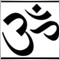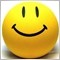# ordermodify at Specific time72

Hi, sir

I are not familiar with English.Please understand me

------------------------------------------------------------

I want to ordermodify at Specific time

for example

evry four hour,, in other word,, evry new H4 candle,,

Hour() = 4, 8, 12, 16, 20, 0.

When i use the function Hour() ,  can i get result i want ?

How can i do?5299

 Use TimeHour( datetime time)
 Sample:``` int h=TimeHour(TimeCurrent()); ```2092

kjwoong001:

Hi, sir

I are not familiar with English.Please understand me

------------------------------------------------------------

I want to ordermodify at Specific time

for example

evry four hour,, in other word,, evry new H4 candle,,

Hour() = 4, 8, 12, 16, 20, 0.

When i use the function Hour() ,  can i get result i want ?

How can i do?

Try some thing like this :

```datetime every_4H;

int init ()
{
every_4H = iTime (Symbol(), PERIOD_H4, 0);
return (0);
}

int start ()
{

if (every_4H != iTime (Symbol(), PERIOD_H4, 0))
{
every_4H != iTime (Symbol(), PERIOD_H4, 0);
//--- OrderModify ();
}

return (0);
}```72

Oh,,!

Thank you for all,, ^.^

I will try that codes

again thank you~